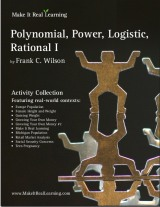GIVEAWAY! Win a Math Mammoth bundle or a grade level of your choice (13 winners!). Take part here. The giveaway runs through Sep 30, 2023.
^
You are here: HomeMake It Real Learning → Polynomial, Power, Logistic, Rational Functions I

# Make It Real Learning Polynomial, Power, Logistic, Rational Functions I - real-life math workbook46 pages
(10 activities)

Free Sample (PDF):
Teen Pregnancy: Investigating Cubic Functions

## Introduction

The Make It Real Learning Polynomial, Power, Logistic, Rational Functions I workbook focuses on real-world situations that may be effectively analyzed using polynomial, power, logistic, and rational functions. This book is suitable for high school or college level algebra 2 or precalculus courses.

From monitoring changes in height and weight to forecasting the population of Michigan, learners get to use mathematics in meaningful ways. Each activity integrates real world information, companies, and issues (e.g. Europe, Michigan, Social Security, teen pregnancy, gaining weight, growing money), not just "realistic" data.

There are multiple ways to use the activities of the book in a teaching environment. The activities are an excellent tool for stimulating mathematical discussions in a small group setting. Due to the challenging nature of each activity, group members are motivated to brainstorm problem solving strategies together. The interesting real world contexts motivate them to want to solve the problems. The activities may also be used for individual projects and class-wide discussions.

As a ready-resource for teachers, the workbook also includes completely worked-out solutions for each activity. To make it easier for teachers to assess student work, the solutions are included on a duplicate copy of each activity.

## Polynomial, Power, Logistic, Rational Functions I Activity Objectives

Activity TitleMathematical Objectives
Europe Population:
Determine which function type best fits a data set
Use regression to find quadratic and cubic function models
Graph polynomial functions
Calculate first and second differences of a data set
Female Height and Weight:
Using Power Function Models
Determine which function type best fits a scatter plot
Use regression to find a power function model
Convert from kilograms to pounds
Convert from centimeters to inches
Gaining Weight:
Using Logistic Function Models
Graph a logistic function
Estimate an inflection point from a graph
Interpret the real-world meaning of an inflection point
Working with Rules of Exponents
Multiply a number by a percent
Divide one number by another
Use rules of exponents to compare two exponential expressions
Working with Rules of Exponents
Multiply a number by a percent
Divide one number by another
Use rules of exponents to compare two exponential expressions
Make It Real Learning:
Working with Function Models
Create a linear model from a verbal description
Create a rational function model
Evaluate linear and rational functions
Michigan Population:
Modeling with Cubic Functions
Determine which function type best fits a data set
Use regression to find a cubic function model
Use a function model to forecast unknown results
Retail Market Analysis:
Working with Rational Functions
Use regression to find a quadratic model
Create a rational function
Determine the practical domain for a function
Evaluate a rational function at a given value
Social Security Concerns: Using
Regression and Rational Functions
Use regression to find linear and quadratic models
Create and graph a rational function model
Teen Pregnancy:
Investigating Cubic Functions
Choose a mathematical model for a given scatter plot
Use technology to find the point of intersection of two graphs
Use a polynomial model to forecast unknown results

Bundle specials!

Activity Library, Volume I. Price: \$39.99
Includes 11 Make It Real Learning activity workbooks for the price of 8! You get three workbooks for free!

Activity Library, Volume II. Price: \$39.99
Includes 11 Make It Real Learning activity workbooks for the price of 8! You get three workbooks for free!

States by the Numbers Bundle. Price: \$19.99
Inludes all 50 States by the Numbers workbooks at a whopping 87% discount!
(Normal price would be 50 × \$2.99 = \$149.50)

By purchasing any of the Make It Real Learning workbooks, permission IS granted for the teacher (or parent) to reproduce this material to be used with his/her students in a teaching situation; not for commercial resale. However, you are not permitted to share the material with another teacher.

In other words, you are permitted to make copies for the students/children you are teaching, but not for other teachers' usage.

WAIT!

Receive my monthly collection of math tips & resources directly in your inbox — and get a FREE Math Mammoth book!You can unsubscribe at any time.

### Math Mammoth Tour

Confused about the different options? Take a virtual email tour around Math Mammoth! You'll receive:

An initial email to download your GIFT of over 400 free worksheets and sample pages from my books. Six other "TOURSTOP" emails that explain the important things and commonly asked questions concerning Math Mammoth curriculum. (Find out the differences between all these different-colored series!)

This way, you'll have time to digest the information over one or two weeks, plus an opportunity to ask me personally about the curriculum.
A monthly collection of math teaching tips & Math Mammoth updates (unsubscribe any time)### "Mini" Math Teaching Course

This is a little "virtual" 2-week course, where you will receive emails on important topics on teaching math, including:

- How to help a student who is behind
- Troubles with word problems
- Teaching multiplication tables
- Why fractions are so difficult
- The value of mistakes
- Should you use timed tests
- And more!

A GIFT of over 400 free worksheets and sample pages from my books right in the very beginning.A monthly collection of math teaching tips & Math Mammoth updates (unsubscribe any time)
Enter your email to receive math teaching tips, resources, Math Mammoth news & sales, humor, and more! I tend to send out these tips about once monthly, near the beginning of the month, but occasionally you may hear from me twice per month (and sometimes less often).• A GIFT of over 400 free worksheets and sample pages from my books.